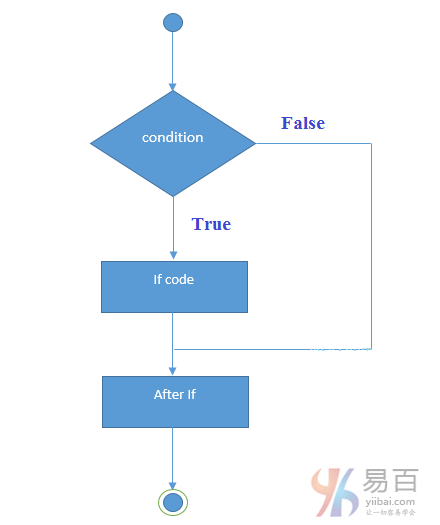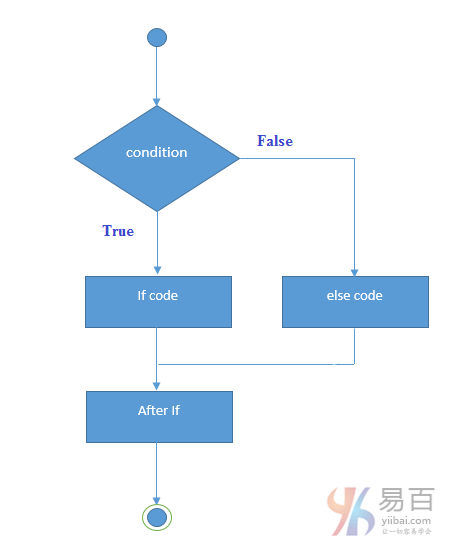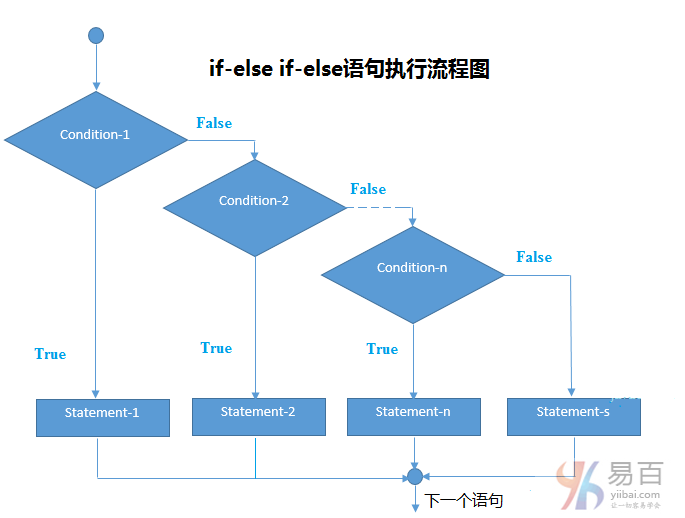# Java if/else语句

Java if语句用于测试条件。它检查布尔条件为：`true``false`。 java中有各种类型的if语句，它们分别如下：

• if语句
• if-else语句
• 嵌套if语句
• if-else-if语句

## Java if语句

Java语言中的`if`语句用于测试条件。如果条件为`true`，则执行`if`语句块。

` `if(condition){      // if 语句块 => code to be executed. } ``
Java### 1. 示例

` `public class IfExample {     public static void main(String[] args) {         int age = 20;         if (age > 18) {             System.out.print("Age is greater than 18");         }     } } ``
Java

` `Age is greater than 18 ``
Java

## Java if-else语句

Java `if-else`语句也用于测试条件。如果`if`条件为真(`true`)它执行`if`块中的代码，否则执行`else`块中的代码。

` `if(condition){       //code if condition is true   }else{       //code if condition is false   } ``
Java` `public class IfElseExample {     public static void main(String[] args) {         int number = 13;         if (number % 2 == 0) {             System.out.println("这是一个偶数");         } else {             System.out.println("这是一个奇数");         }     } } ``
Java

` `这是一个奇数 ``
Java

## Java if-else-if语句

Java编程中的`if-else-if`语句是从多个语句中执行一个条件。

` `if(condition1){       //code to be executed if condition1 is true   }else if(condition2){       //code to be executed if condition2 is true   }else if(condition3){       //code to be executed if condition3 is true   }   ...   else{       //code to be executed if all the conditions are false   } ``
Java` `public class IfElseIfExample {     public static void main(String[] args) {         int marks = 65;          if (marks < 50) {             System.out.println("fail");         } else if (marks >= 50 && marks < 60) {             System.out.println("D grade");         } else if (marks >= 60 && marks < 70) {             System.out.println("C grade");         } else if (marks >= 70 && marks < 80) {             System.out.println("B grade");         } else if (marks >= 80 && marks < 90) {             System.out.println("A grade");         } else if (marks >= 90 && marks < 100) {             System.out.println("A+ grade");         } else {             System.out.println("Invalid!");         }     } } ``
Java

` `C grade``

## 相关资讯

• #### 学堂故事

•尚学堂微博尚学堂订阅号尚学堂服务号

• 北京校区
• 西安校区
• 山西校区
• 武汉校区
• 深圳校区
• 上海校区
• 广州校区
• 保定招生办
• 黑龙江项目办